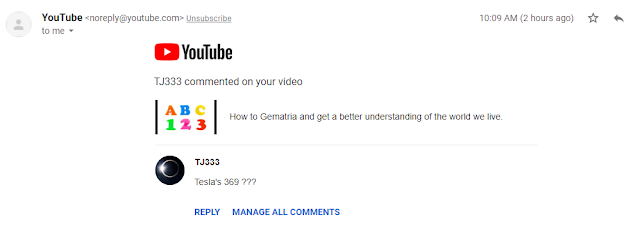## Saturday, December 7, 2019

### Comment on my 11 video this morning about Tesla and 369(The KEY)I wake up this morning to a comment on 2 of my videos. One of them of course is the video I made in regards to the number "11" that I just blogged about last night.
It's also interesting as I mentioned "The Key" and this person keeps asking me about Tesla saying the Key to the universe is 369.
One thing that has always stuck out to me about 369.
1+1+1=3
2+2+2=6
3+3+3=9
4+4+4=12=1+2=3
5+5+5=15=1+5=6
6+6+6=18=1+8=9
7+7+7=21=2+1=3
8+8+8=24=2=4=6
9+9+9=27=2+7=9
By tripling each digit in order and reducing to numerology in order it gives you the 369 pattern.
3+6+9=18+1+8=9
TJ 3+3+3=9
2+1+3+3+3=12=1+2=3

Ironically the word "Hermeticism"=369(Jewish) which is something that has been important to what I have been documenting about lately too.

Maybe I am mistaken and the Key is actually 9 or 11....The 177 and Aurora just showing me to pay attention to this. I mean I was documenting about the 11's with 9/11 when this synchronicity started last night.

It's no coincidence Tesla(band) remade the song "Signs, Signs, Everywhere there's signs" either.